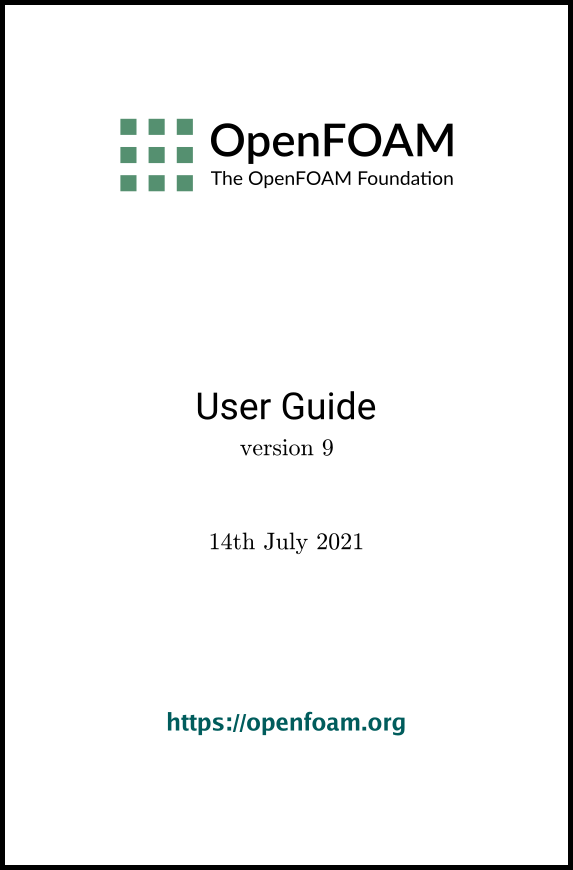## 6.2Post-processing command line interface (CLI)

Post-processing is provided directly within OpenFOAM through the command line including data processing, sampling (e.g. probes, graph plotting) visualisation, case control and run-time I/O. Functionality can be executed by:

• conventional post-processing, a data processing activity that occurs after a simulation has run;
• run-time processing, data processing that is performed during the running of a simulation.

Both approaches have advantages. Conventional post-processing allows the user to choose how to analyse data after the results are obtained. Run-time processing oﬀers greater ﬂexibility because it has access to all the data in the database of the run at all times, rather than just the data written during the simulation. It also allows the user to monitor processed data during a simulation and provides a greater level of convenience because the processed results can be available immediately to the user when the simulation ends.

There are 3 methods of post-processing that cover the options described above

• Every solver, e.g. simpleFoam can be conﬁgured to include run-time processing.
• The postProcess utility provides conventional post-processing of data written by a simulation.
• Every solver can be run with the -postProcess option, which only executes post-processing, but with additional access to data available on the database for the particular solver.

### 6.2.1Post-processing functionality

All modes of post-processing access the same functionality implemented in OpenFOAM in the function object framework. Function objects can be listed by running a solver with the -listFunctionObjects option, e.g.

simpleFoam -listFunctionObjects
The list represents the underlying post-processing functionality. Almost all the functionality is packaged into a set of conﬁgured tools that are conveniently integrated within the post-processing CLI. Those tools are located in \$FOAM_ETC/caseDicts/postProcessing and are listed by running postProcess with the -list option.

postProcess -list
This produces a list of tools that are described in the following sections.

#### 6.2.1.1Field calculation

age
Calculates and writes out the time taken for a particle to travel from an inlet to the location.
components
Writes the component scalar ﬁelds (e.g. Ux, Uy, Uz) of a ﬁeld (e.g. U).
CourantNo
Calculates the Courant Number ﬁeld from the ﬂux ﬁeld.
ddt
Calculates the Eulerian time derivative of a ﬁeld.
div
Calculates the divergence of a ﬁeld.
enstrophy
Calculates the enstrophy of the velocity ﬁeld.
ﬁeldAverage
Calculates and writes the time averages of a given list of ﬁelds.
ﬂowType
Calculates and writes the flowType of velocity ﬁeld where: -1 = rotational ﬂow; 0 = simple shear ﬂow; +1 = planar extensional ﬂow.
Calculates the gradient of a ﬁeld.
Lambda2
Calculates and writes the second largest eigenvalue of the sum of the square of the symmetrical and anti-symmetrical parts of the velocity gradient tensor.
log
Calculates the natural logarithm of the speciﬁed scalar ﬁeld.
MachNo
Calculates the Mach Number ﬁeld from the velocity ﬁeld.
mag
Calculates the magnitude of a ﬁeld.
magSqr
Calculates the magnitude-squared of a ﬁeld.
PecletNo
Calculates the Peclet Number ﬁeld from the ﬂux ﬁeld.
phaseMap
Writes the phase-fraction map ﬁeld alpha.map with incremental value ranges for each phase e.g., with values 0 for water, 1 for air, 2 for oil, etc.
Q
Calculates the second invariant of the velocity gradient tensor.
randomise
Adds a random component to a ﬁeld, with a speciﬁed perturbation magnitude.
scale
Multiplies a ﬁeld by a scale factor
shearStress
Calculates the shear stress, outputting the data as a volSymmTensorField.
streamFunction
Writes the stream-function pointScalarField, calculated from the speciﬁed ﬂux surfaceScalarField.
totalEnthalpy
Calculates and writes the total enthalpy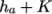as the volScalarField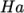.
turbulenceFields
Calculates speciﬁed turbulence ﬁelds and stores it on the database.
turbulenceIntensity
Calculates and writes the turbulence intensity ﬁeld I.
vorticity
Calculates the vorticity ﬁeld, i.e. the curl of the velocity ﬁeld.
wallHeatFlux
Calculates the heat ﬂux at wall patches, outputting the data as a volVectorField.
wallHeatTransferCoeﬀ
Calculates the estimated incompressible ﬂow heat transfer coeﬃcient at wall patches, outputting the data as a volScalarField.
wallShearStress
Calculates the shear stress at wall patches, outputting the data as a volVectorField.
writeCellCentres
Writes the cell-centres volVectorField and the three component ﬁelds as volScalarFields; useful for post-processing thresholding.
writeCellVolumes
Writes the cell-volumes volScalarField
writeVTK
Writes out speciﬁed objects in VTK format, e.g. ﬁelds, stored on the case database.
yPlus
Calculates the turbulence y+, outputting the data as a yPlus ﬁeld.

#### 6.2.1.2Field operations

divide
From the ﬁrst ﬁeld, divide the remaining ﬁelds in the list.
multiply
Multiply a list of ﬁelds.
subtract
From the ﬁrst ﬁeld, subtracts the remaining ﬁelds in the list.
uniform
Create a uniform ﬁeld.

#### 6.2.1.3Forces and force coeﬃcients

forceCoeﬀsCompressible
Calculates lift, drag and moment coeﬃcients by summing forces on speciﬁed patches for a case where the solver is compressible (pressure is in units M/(LTˆ2), e.g. Pa).
forceCoeﬀsIncompressible
Calculates lift, drag and moment coeﬃcients by summing forces on speciﬁed patches for a case where the solver is incompressible (pressure is kinematic, e.g. mˆ2/sˆ2).
forcesCompressible
Calculates pressure and viscous forces over speciﬁed patches for a case where the solver is compressible (pressure is in units M/(LTˆ2), e.g. Pa).
forcesIncompressible
Calculates pressure and viscous forces over speciﬁed patches for a case where the solver is incompressible (pressure is kinematic, e.g. mˆ2/sˆ2).
phaseForces
Calculates the blended interfacial forces acting on a given phase, i.e. drag, virtual mass, lift, wall-lubrication and turbulent dispersion. Note that it works only in solver post-processing mode and in combination with multiphaseEulerFoam. For a simulation involving more than two phases, the accumulated force is calculated by looping over all phasePairs the phase is a part of.

#### 6.2.1.4Sampling for graph plotting

graphCell
Writes graph data for speciﬁed ﬁelds along a line, speciﬁed by start and end points. One graph point is generated in each cell that the line intersects.
graphUniform
Writes graph data for speciﬁed ﬁelds along a line, speciﬁed by start and end points. A speciﬁed number of graph points are used, distributed uniformly along the line.

#### 6.2.1.5Lagrangian data

dsmcFields
Calculate intensive ﬁelds UMean, translationalT, internalT, overallT from averaged extensive ﬁelds from a DSMC calculation.

#### 6.2.1.6Monitoring minima and maxima

cellMax
Writes out the maximum cell value for one or more ﬁelds.
cellMaxMag
Writes out the maximum cell value magnitude for one or more ﬁelds.
cellMin
Writes out the minimum cell value for one or more ﬁelds.
cellMinMag
Writes out the maximum cell value magnitude for one or more ﬁelds.

#### 6.2.1.7Numerical data

residuals
For speciﬁed ﬁelds, writes out the initial residuals for the ﬁrst solution of each time step; for non-scalar ﬁelds (e.g. vectors), writes the largest of the residuals for each component (e.g. x, y, z).

#### 6.2.1.8Control

stopAtClockTime
Stops the run when the speciﬁed clock time in second has been reached and optionally write results before stopping.
stopAtFile
Stops the run when the ﬁle stop is created in the case directory.
time
Writes run time, CPU time and clock time and optionally the CPU and clock times per time step.
timeStep
Writes the time step to a ﬁle for monitoring.
writeObjects
Writes out speciﬁed objects, e.g. ﬁelds, stored on the case database.

#### 6.2.1.9Pressure tools

staticPressureIncompressible
Calculates the pressure ﬁeld in units M/(LTˆ2) (e.g. Pa) from kinematic pressure by scaling by a speciﬁed density.
totalPressureCompressible
Calculates the total pressure ﬁeld for a case where the solver is compressible (pressure is in units M/(LTˆ2), e.g. Pa).
totalPressureIncompressible
Calculates the total pressure ﬁeld for a case where the solver is incompressible (pressure is kinematic, e.g. mˆ2/sˆ2).

#### 6.2.1.10Combustion

Qdot
Calculates and outputs the heat release rate for the current combustion model.
XiReactionRate
Writes the turbulent ﬂame-speed and reaction-rate volScalarFields for the Xi-based combustion models.

#### 6.2.1.11Probes

boundaryProbes
Writes out values of ﬁelds at a cloud of points, interpolated to speciﬁed boundary patches.
interfaceHeight
Reports the height of the interface above a set of locations. For each location, it writes the vertical distance of the interface above both the location and the lowest boundary. It also writes the point on the interface from which these heights are computed.
internalProbes
Writes out values of ﬁelds interpolated to a speciﬁed cloud of points.
probes
Writes out values of ﬁelds from cells nearest to speciﬁed locations.

#### 6.2.1.12Surface region

faceZoneAverage
Calculates the average value of one or more ﬁelds on a faceZone.
faceZoneFlowRate
Calculates the ﬂow rate through a speciﬁed face zone by summing the ﬂux on patch faces. For solvers where the ﬂux is volumetric, the ﬂow rate is volumetric; where ﬂux is mass ﬂux, the ﬂow rate is mass ﬂow rate.
patchAverage
Calculates the average value of one or more ﬁelds on a patch.
patchDiﬀerence
Calculates the diﬀerence between the average values of ﬁelds on two speciﬁed patches. Calculates the average value of one or more ﬁelds on a patch.
patchFlowRate
Calculates the ﬂow rate through a speciﬁed patch by summing the ﬂux on patch faces. For solvers where the ﬂux is volumetric, the ﬂow rate is volumetric; where ﬂux is mass ﬂux, the ﬂow rate is mass ﬂow rate.
patchIntegrate
Calculates the surface integral of one or more ﬁelds on a patch.
triSurfaceDiﬀerence
Calculates the diﬀerence between the average values of ﬁelds on two speciﬁed triangulated surfaces.
triSurfaceVolumetricFlowRate
Calculates volumetric ﬂow rate through a speciﬁed triangulated surface by interpolating velocity onto the triangles and integrating over the surface area. Triangles need to be small (¡= cell size) for an accurate result.

#### 6.2.1.13‘Pluggable’ solvers

particles
Tracks a cloud of parcels driven by the ﬂow of the continuous phase.
phaseScalarTransport
Solves a transport equation for a scalar ﬁeld within one phase of a multiphase simulation.
scalarTransport
Solves a transport equation for a scalar ﬁeld.

#### 6.2.1.14Visualisation tools

cutPlaneSurface
Writes out cut-plane surface ﬁles with interpolated ﬁeld data in VTK format.
isoSurface
Writes out iso-surface ﬁles with interpolated ﬁeld data in VTK format.
patchSurface
Writes out patch surface ﬁles with interpolated ﬁeld data in VTK format.
streamlinesLine
Writes out ﬁles of stream lines with interpolated ﬁeld data in VTK format, with initial points uniformly distributed along a line.
streamlinesPatch
Writes out ﬁles of stream lines with interpolated ﬁeld data in VTK format, with initial points randomly selected within a patch.
streamlinesPoints
Writes out ﬁles of stream lines with interpolated ﬁeld data in VTK format, with speciﬁed initial points.
streamlinesSphere
Writes out ﬁles of stream lines with interpolated ﬁeld data in VTK format, with initial points randomly selected within a sphere.

### 6.2.2Run-time data processing

When a user wishes to process data during a simulation, they need to conﬁgure the case accordingly. The conﬁguration process is as follows, using an example of monitoring ﬂow rate at an outlet patch named outlet.

Firstly, the user should include the ﬂowRatePatch function in functions sub-dictionary in the case controlDict ﬁle, using the #includeFunc directive.

functions
{
#includeFunc  flowRatePatch
... other function objects here ...
}
That will include the functionality in the ﬂowRatePatch conﬁguration ﬁle, located in the directory hierarchy beginning with \$FOAM_ETC/caseDicts/postProcessing.

The conﬁguration of ﬂowRatePatch requires the name of the patch to be supplied. Option 1 for doing this is that the user copies the ﬂowRatePatch ﬁle into their case system directory. The foamGet script copies the ﬁle conveniently, e.g.

foamGet flowRatePatch
The patch name can be edited in the copied ﬁle to be outlet. When the solver is run, it will pick up an included function in the local case system directory, in precedence over \$FOAM_ETC/caseDicts/postProcessing. The ﬂow rate through the patch will be calculated and written out into a ﬁle within a directory named postProcessing.

Option 2 for specifying the patch name is to provide the name as an argument to the ﬂowRatePatch in the #includeFunc directive, using the syntax keyword=entry.

functions
{
#includeFunc  flowRatePatch(patch=outlet)
... other function objects here ...
}

In the case where the keyword is field or fields, only the entry is needed when specifying an argument to a function. For example, if the user wanted to calculate and write out the magnitude of velocity into time directories during a simulation they could simply add the following to the functions sub-dictionary in controlDict.

functions
{
#includeFunc  mag(U)
... other function objects here ...
}
This works because the function’s argument U is represented by the keyword field, see \$FOAM_ETC/caseDicts/postProcessing/ﬁelds/mag.

Some functions require the setting of many parameters, e.g. to calculate forces and generate elements for visualisation, etc. For those functions, it is more reliable and convenient to copy and conﬁgure the function using option 1 (above) rather than through arguments.

### 6.2.3The postProcess utility

The user can execute post-processing functions after the simulation is complete using the postProcess utility. Let us illustrate the use of postProcess using the pitzDaily case from the tutorials directory. The case can be copied, e.g. into the user’s run directory; the user can then go into the case directory, generate the mesh with blockMesh and then run simpleFoam

run
cp -r \$FOAM_TUTORIALS/incompressible/simpleFoam/pitzDaily .
cd pitzDaily
blockMesh
simpleFoam
Now the user can run execute post-processing functions with postProcess. The -help option provides a summary of its use.

postProcess -help
Simple functions like mag can be executed using the -func option; text on the command line generally needs to be quoted ("") if it contains punctuation characters.

postProcess -func "mag(U)"
This operation calculates and writes the ﬁeld of magnitude of velocity into a ﬁle named mag(U) in each time directory. Similarly, the ﬂowRatePatch example can be executed using postProcess.

postProcess -func "flowRatePatch(name=outlet)"
Let us say the user now wants to calculate total pressure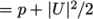for incompressible ﬂow with kinematic pressure,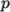. The function is available, named totalPressureIncompressible, which the user could attempt ﬁrst to run as follows.

postProcess -func totalPressureIncompressible
This returns the following error message.

--> FOAM Warning : functionObject pressure: Cannot find required field p
The error message is telling the user that the pressure ﬁeld p is not loaded; the same is true of the velocity ﬁeld U. For the function to work, both ﬁelds can be loaded as comma separated arguments.

postProcess -func "totalPressureIncompressible(p,U)"
Alternatively the user can load a space separated list of ﬁelds using the -fields option, which the function can access.

postProcess -fields "(p U)" -func totalPressureIncompressible
Both options work eﬀectively because the pressure and velocity data is available directly from the ﬁles, p and U.

### 6.2.4Solver post-processing

A more complex example is calculating wall shear stress using the wallShearStress function.

postProcess -fields "(p U)" -func wallShearStress

--> FOAM FATAL ERROR:
Unable to find turbulence model in the database
The message is telling us that the postProcess utility has not constructed the necessary models that the solver, simpleFoam, used when running the simulation, i.e. a turbulence model. This is a situation where we need to post-process (as opposed to run-time process) using the solver with the -postProcess option so that the modelling will be available that the post-processing function needs. Help for this operation can be printed with the following command.

simpleFoam -postProcess -help
It can be seen that the options for a solver with -postProcess are the same as when running postProcess utility. This means that the -func option can be used to execute the wallShearStress function eﬀectively.

simpleFoam -postProcess -func wallShearStress
Note that no ﬁelds need to be supplied, either by function arguments ”(p,U)” or using ”-fields (p U)”, because simpleFoam itself constructs and stores the required ﬁelds. Functions can also be selected by the #includeFunc directive in functions in the controlDict ﬁle, instead of the -func option.
OpenFOAM v9 User Guide - 6.2 Post-processing command line interface (CLI)